# Javascript Searching Algorithms

Learn Linear search and binary search in javascript
javascriptAlgorithms
30-September-2020

# Javascript Searching Algorithms

In This Post, I will try to cover search in javascript. It is not going to be any complicated searching algorithm but rather simpler algorithms that are commonly used. Javascript provides several search methods like `indexOf` `includes` `find` and many others. Our Focus here would be on how to implement our version of these methods.

We will cover two algorithms in this post Linear Search and Binary Search.

First thing first the coding environment. You Can use any editor you want local or online. But here I will be using google chrome snippets. Our code will be plain javascript therefore we don't need any fancy environment. If you want to follow along head on to google chrome's dev tools `ctrl + shift + I` . Click on the sources tab and from the left navigator select snippets. Create new snippets and name it linearSearch.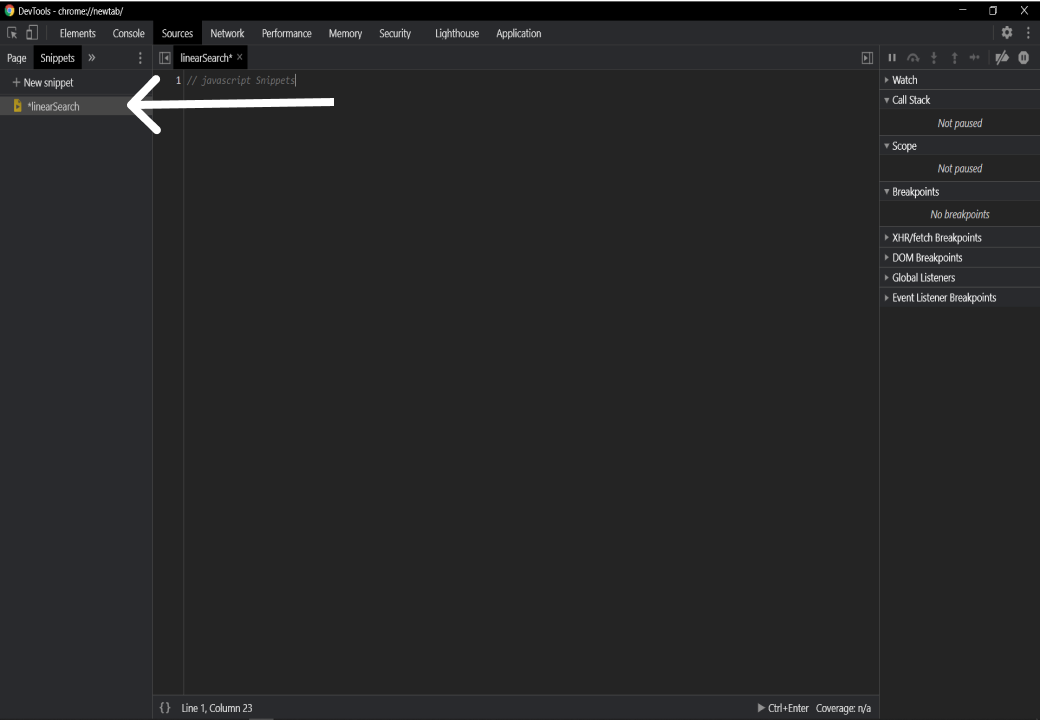we can use `ctrl + Enter` to run the code as you can see at the bottom of the above image. Now that that's out of the way lets begin.

## Linear Search

All the javascript search methods like `find, indexOf` etc. are using Linear Search. This is the simplest way of searching. Given an array, we look at every element to find what we are looking for. We check one item at a time starting from the beginning of the array or end of the array. Let's say we have a list

`const list = [12, 45, 48, 5, 451, 2,34 ,43,54,66 ]`

we want to search for `2`. The data is not sorted in this array so the best approach would be to loop through every item in the array and check if the current iteration is equal to `2`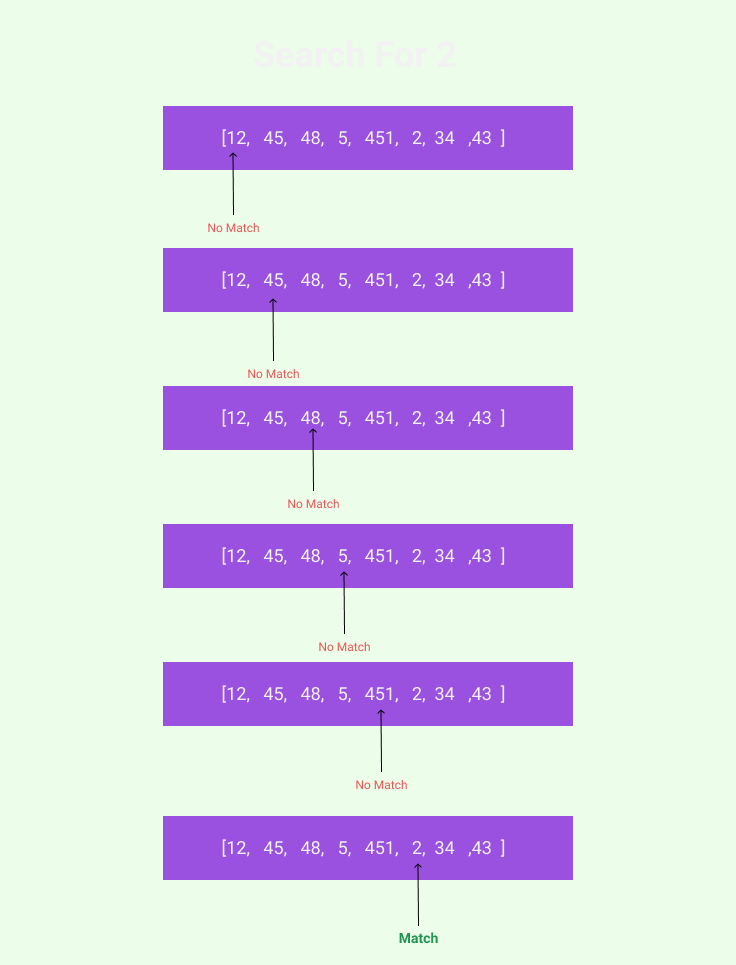pretty Simple Right.

Lets Code this. How are we going to approach this? Let's Break it down into pieces.

• We will write a function named you guessed it `linearSearch`. That function will accept two arguments. an array and a value.
• Inside that function, we will loop through the entire array and check if current item is equal to value.
• If The value is found we will return the `index` of that value otherwise we will return `false` or `-1`

Step One

A function that will accept two arguments

``````1
var linearSearch = (list, value) => {};``````

If you are using google chrome Snippets and want to use `const` or `let` Please use `let` because if you use `const` you cannot redeclare the variable and google chrome console will through an error.

Step Two

First, create a `list` and `value`. Two arguments our function needs.

``````1
2
3
4
5
6
let linearSearch = (list, value) => {};

var list = [12, 45, 48, 5, 451, 2, 34, 43, 54, 66];
var value = 2;

linearSearch(list, value); // call the function with arguments``````

Now we will implement the Logic.

``````1
2
3
4
5
6
7
8
9
10
11
12
13
let linearSearch = (list, value) => {
for (let i = 0; i < list.length; i++) {
if (list[i] === value) {
return i;
}
}
return -1;
};

var list = [12, 45, 48, 5, 451, 2, 34, 43, 54, 66];
var value = 2;

linearSearch(list, value); // result should 5``````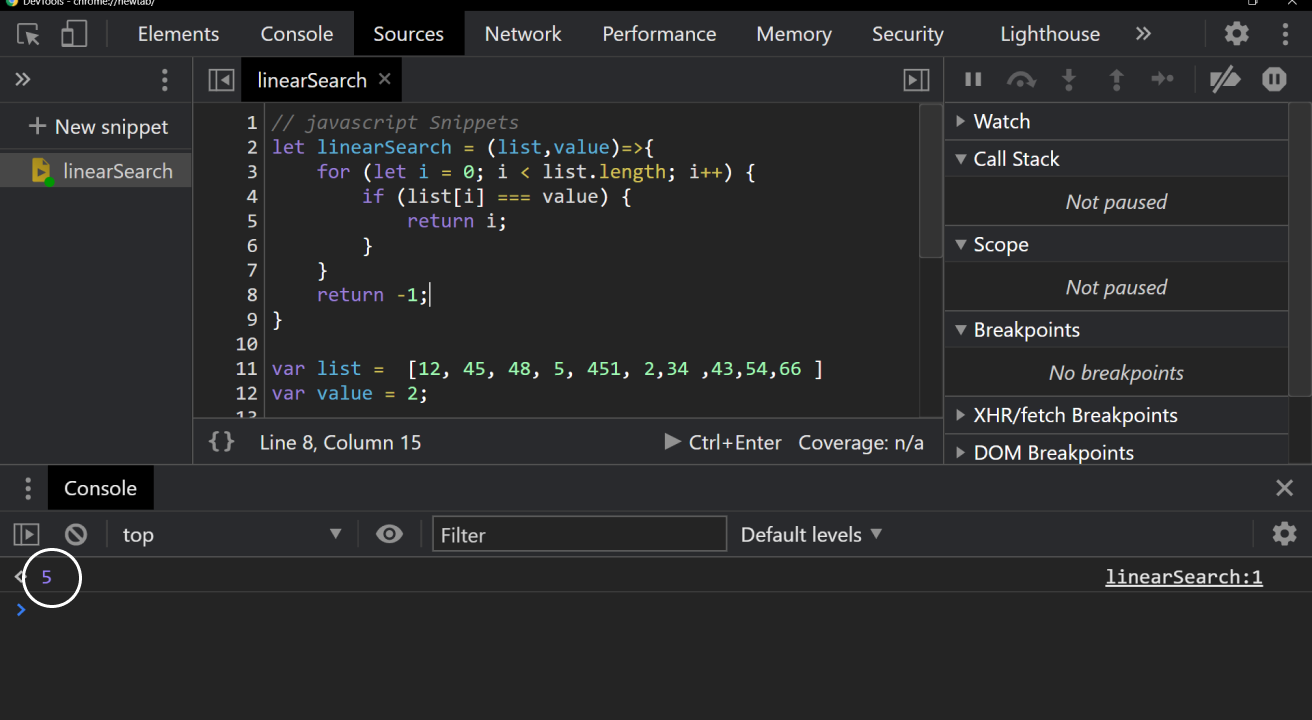Let's try to understand understand what is going on inside the loop

We can refer to an element inside an array as `arr` this will give us the first value and `arr` will give us the second value and so on.

Let see this in action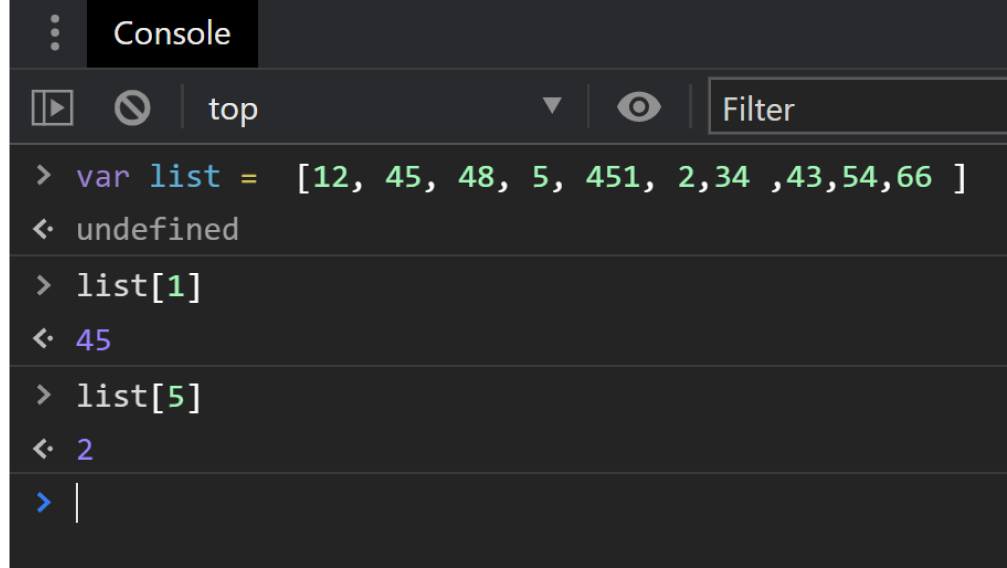in our loop `i` will be incremented from `0` to `9`. on each iteration we will get the value from `list` of that index `list[i]` and compare it with our argument value;

we can confirm this with by`debugger` in our snippet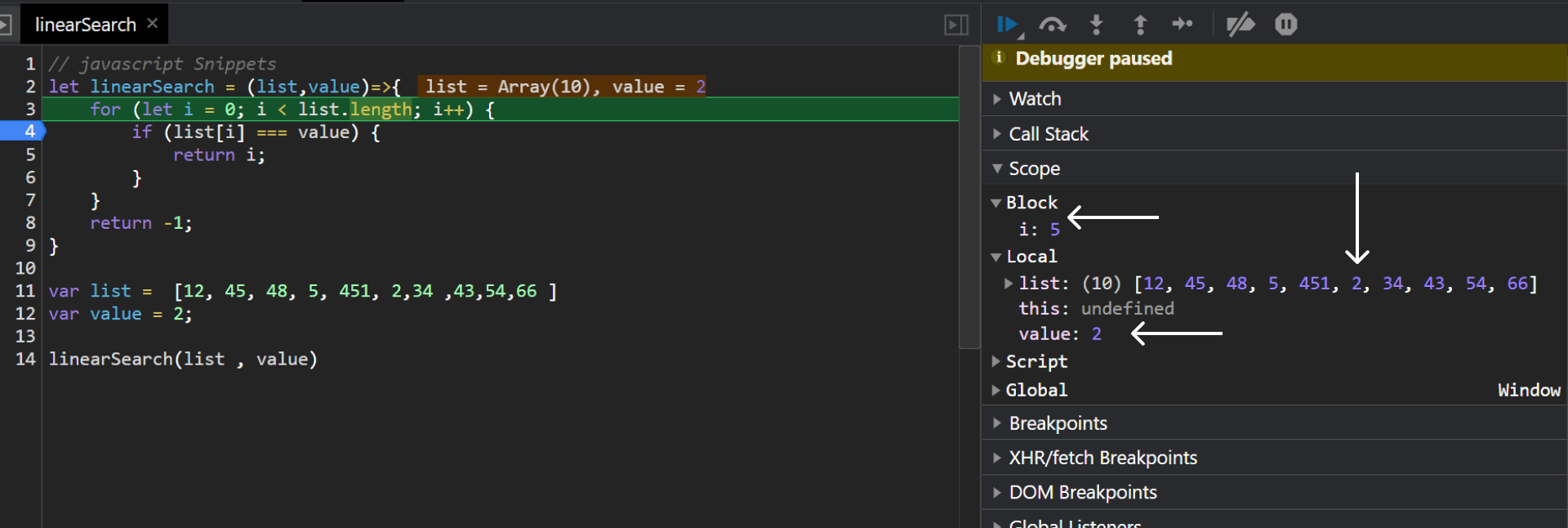I clicked on line 4 to add `debugger`. You can see step by step iteration by pressing `f9`. The above step is the step where we find our match( step 6 with `i = 5`). You can see in the `Block` panel (left side) all the variables we have access to.

I would Suggest you play around with debugger to see the `call Stack` `Block` `local` and `global` scope

We are returning `-1` outside of the loop if we don't find the match.

NOTE: Return `-1` outside of the loop

Final Step

Let's check the condition where value is not in `list`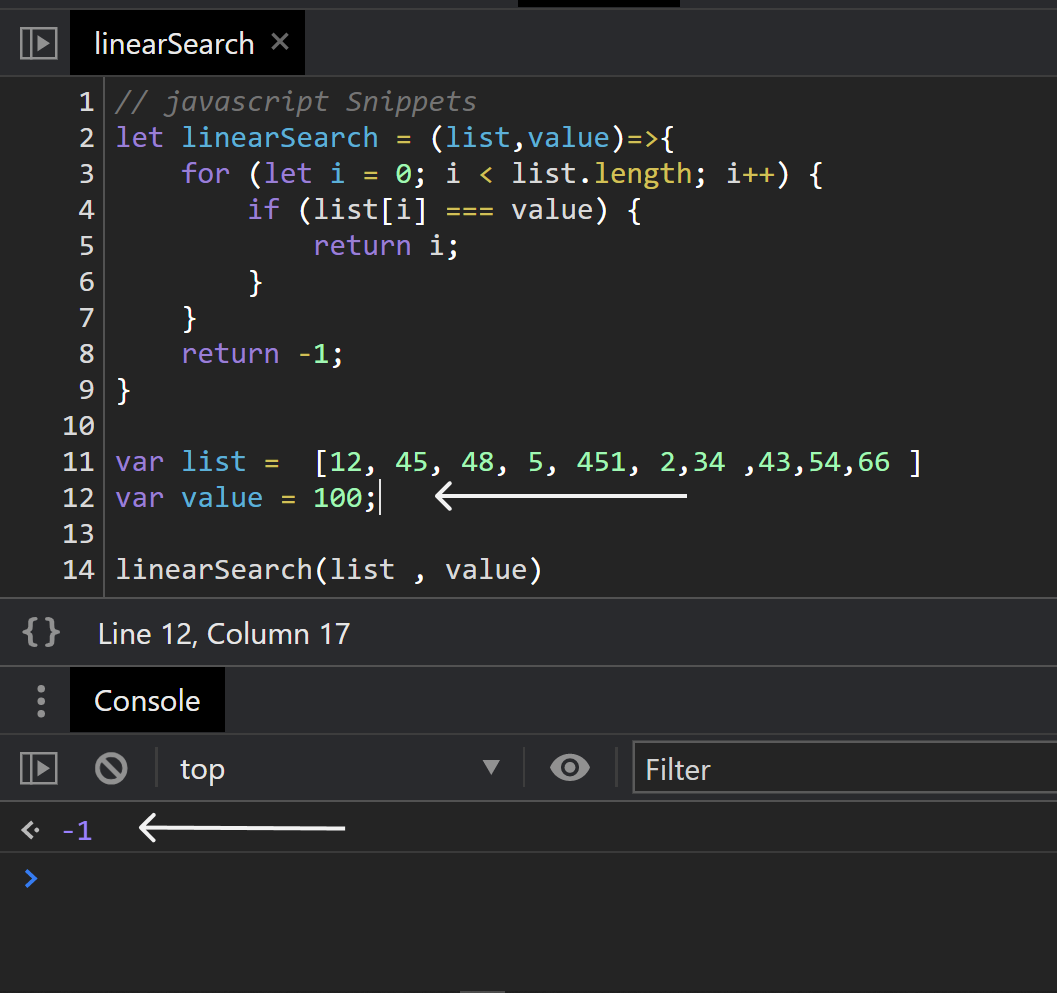Great! It is working

Keep that in mind that array can be sorted or unsorted in linear search The best-case scenario is we will find the item we are looking for immediately and worst-case scenario is that our required item is the last item in the array. For small arrays, it works fine but for large arrays performance might not be ideal.

Let's Move on to Binary Search Now.

## Binary Search

Binary search is a much faster algorithm because of the way it works. At any given point it eliminates half of the array.

But The only caveat is it only works on Sorted arrays.

How it works

Because the array is sorted we pick the middle point of the array. After setting the middle point we will check if the value we are looking for is greater then or less then our midpoint. If The Value is greater then the midpoint that means our value is on the right side of our midpoint so we don't need left (or less then side) so we ditch the left side and look in the right side. We will keep doing that until we find our value.

confused.?

Let's try to visualize this. Define our array first.

``````1
let list = [2, 4, 6, 8, 10, 12, 14, 16, 18, 20, 22, 24, 26, 28, 30];``````

let's say we are looking for `20`

We Need three points `left` , `right` , `middle`

`left = 2`

`right = 30`

mid point could be `14` or `16`. I am going to pick `14`

our mid point is `14` and our value is `20` so we will eliminate the left side which is from `2` to `14`

our arrays would look like this now

``````1
let list = [16, 18, 20, 22, 24, 26, 28, 30];``````

our next mid point will be our value between `22` and `24` we will pick `22` and `left = 16`, `right = 30`

From our mid `(22)`, is our value (`20`) grater or less? It's less than right.? so this time we eliminate items on the right side

our new array should look like this

``````1
let list = [16, 18, 20, 22];``````

mid point `18` left `16` right `22`.

our value is greater than `18`

``````1
let list = [20, 22];``````

`mid point === 20`

Mid point === value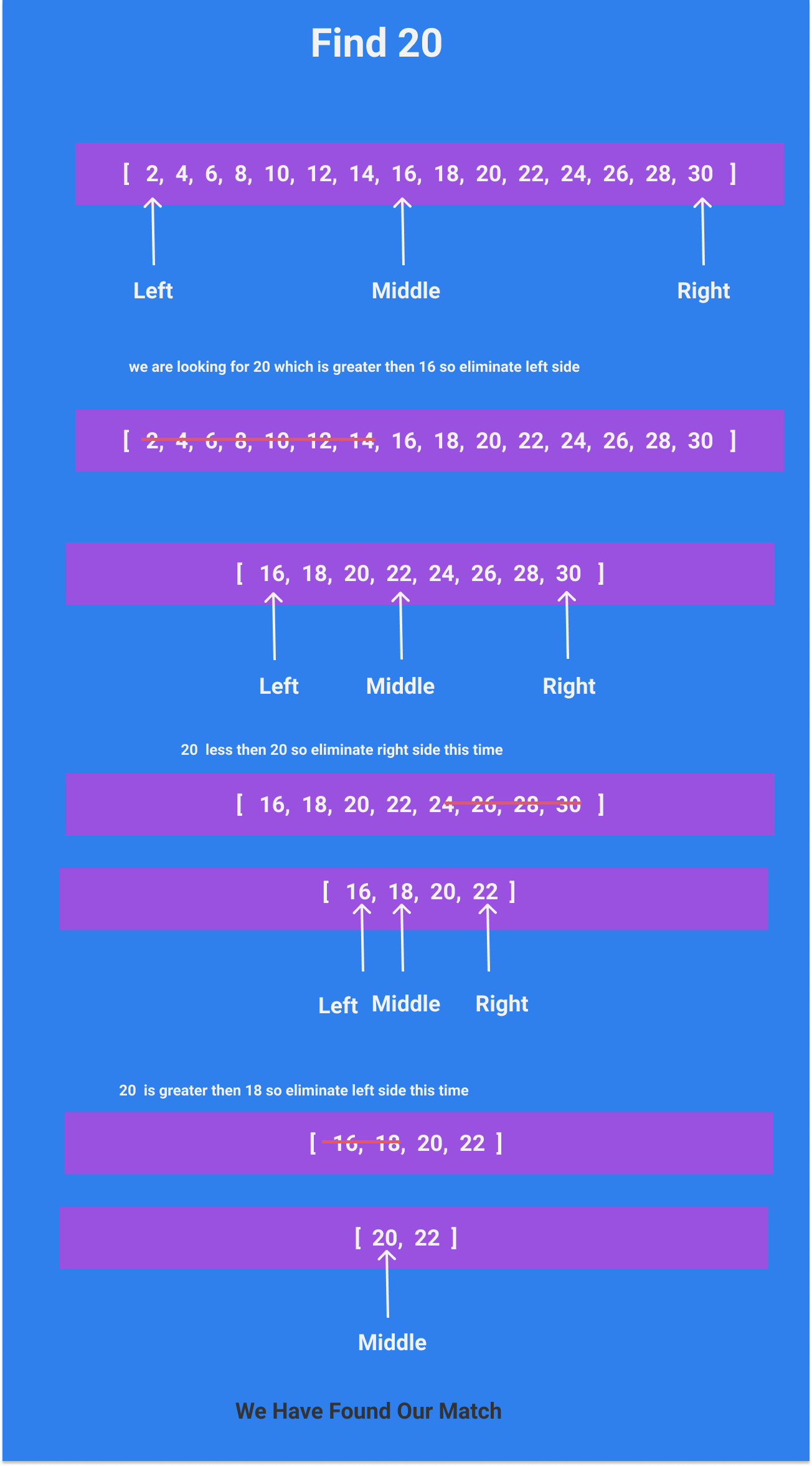In Just Three loops we have found our Value. If We do the same with linear search it would take around 10 loops to find value `20`

binary search is much faster. But it only works in sorted data.

Lets code this. So How Should we approach this? Let's think this through.

• We will write a function that accepts two arguments a sorted array and a value.
• we need Left and Right pointers. So We will create variable `left` whose value will be the first item in our array and the right variable whose value will be the last item in the array
• we also need a middle point which we can get from an average of `left` and `right`
• we will loop until the mid === value
• if we find the value we will return the index if that value
• if the value is too small we will move left pointer up to the previous mid point and recalculate the mid point
• if the value is too large we will move the right pointer down to the mid point and so on and on until we find our value.
• If Value Not found we will return `false` or `-1`

Hwww. That is a lot but let's get through this step by step.

Let's define a function, a sorted array, and a value.

``````1
2
3
4
let BinarySearch = (list, val) => {};

let list = [2, 4, 6, 8, 10, 12, 14, 16, 18, 20, 22, 24, 26, 28, 30];
let val = 20;``````

We Need Three pointers here. `left`, `right`, `mid`

``````1
2
3
let left = 0;
let right = list.length - 1;
let mid = Math.floor((left + right) / 2);``````

`left` is `0` because arrays are zero index so the first item in the array will at `0` index.

`right` again because arrays are zero index so to get the last item we will subtract 1 from its length.

`mid` to calculate average we use this formula `(left + right) / 2`. we don't want decimal number so we use javascript built in method `Math.floor()`. You can also use `Math.ceil()`

to loop through the array we will use while loop

``````1
2
3
4
5
6
7
8
9
10
11
12
13
14
15
16
17
18
19
20
21
22
23
24
let BinarySearch = (list, val) => {
let left = 0;
let right = list.length - 1;
let mid = Math.floor((left + right) / 2);

while (list[mid] !== val && left <= right) {
if (val < list[mid]) {
right = mid - 1;
} else {
left = mid + 1;
}
mid = Math.floor((left + right) / 2);
}
if (list[mid] === val) {
return mid;
} else {
return -1;
}
};
let list = [2, 4, 6, 8, 10, 12, 14, 16, 18, 20, 22, 24, 26, 28, 30];
let val = 20;
// should return 9

BinarySearch(list, val);``````

Scary huh.? Let's Walk this through

First, we will try to understand while loop

``````1
2
3
4
5
6
7
8
while (list[mid] !== val) {
if (val < list[mid]) {
right = mid - 1;
} else {
left = mid + 1;
}
mid = Math.floor((left + right) / 2);
}``````

in first line we are saying loop until the current iteration item is not equal to value.

inside the loop we checking our conditions

if our value (20) is less then the current iteration item that means we need to move the right end towards the middle.

otherwise the value is greater then current iteration item so our left should move towards the middle.

on each iteration, we are recalculating our middle point. The Above code will work fine until we provide false value.

in case of false or no match, we will be in infinite loop. So We need to handle it appropriately.

First of all we want the code to run until `left` is greater than or equal to `right`.

So Modify the above code.

``````1
2
3
4
5
6
7
8
9
while (list[mid] !== val && left <= right) {
// <-- modified
if (val < list[mid]) {
right = mid - 1;
} else {
left = mid + 1;
}
mid = Math.floor((left + right) / 2);
}``````

And Check if our mid point is equal to the value we are looking for then return `mid` otherwise return `-1`

``````1
2
3
4
5
6
7
8
9
10
11
12
13
14
15
while (list[mid] !== val && left <= right) {
if (val < list[mid]) {
right = mid - 1;
} else {
left = mid + 1;
}
mid = Math.floor((left + right) / 2);
}

if (list[mid] === val) {
return mid;
} else {
return -1;
}``````

let's Test this out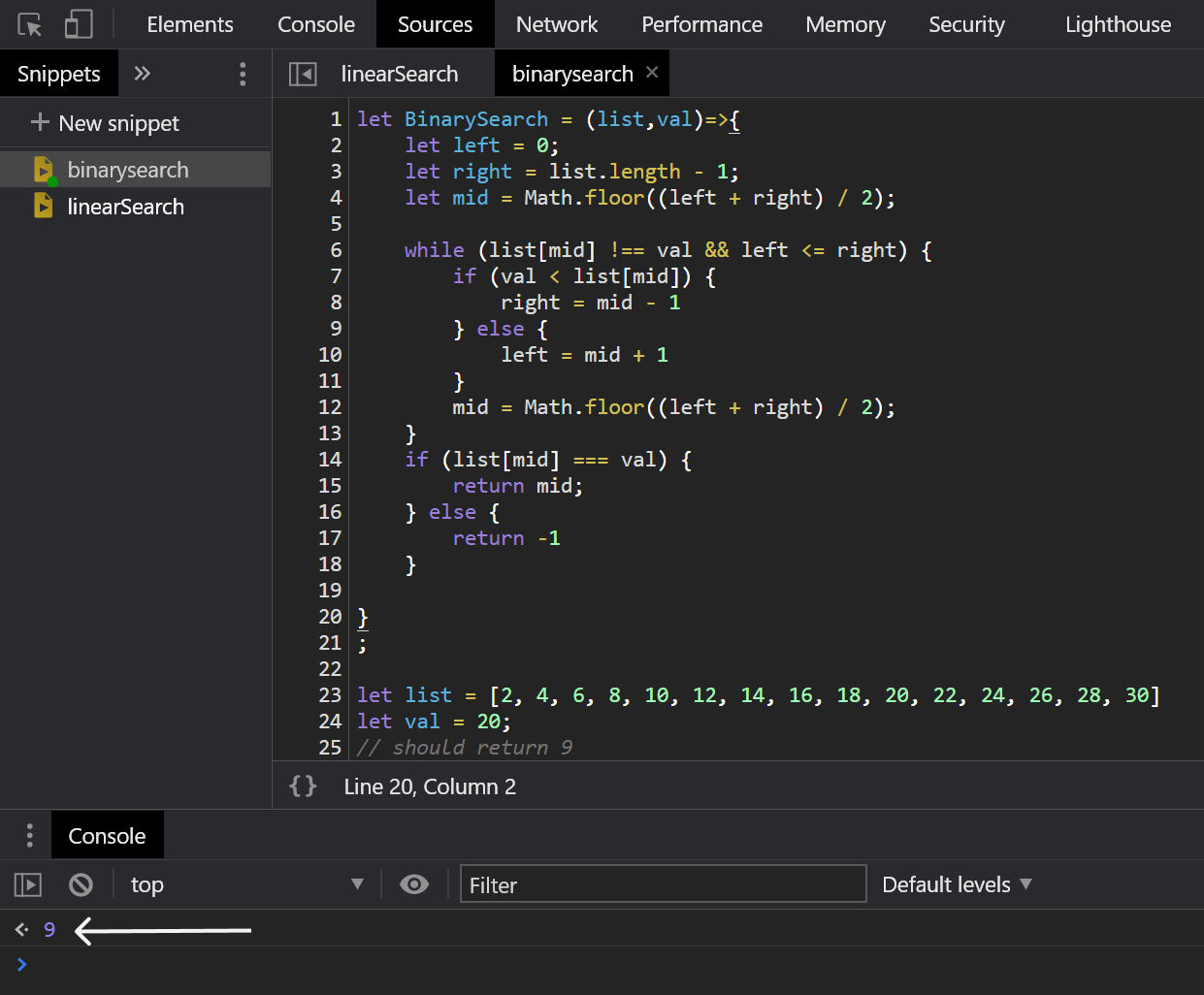With False value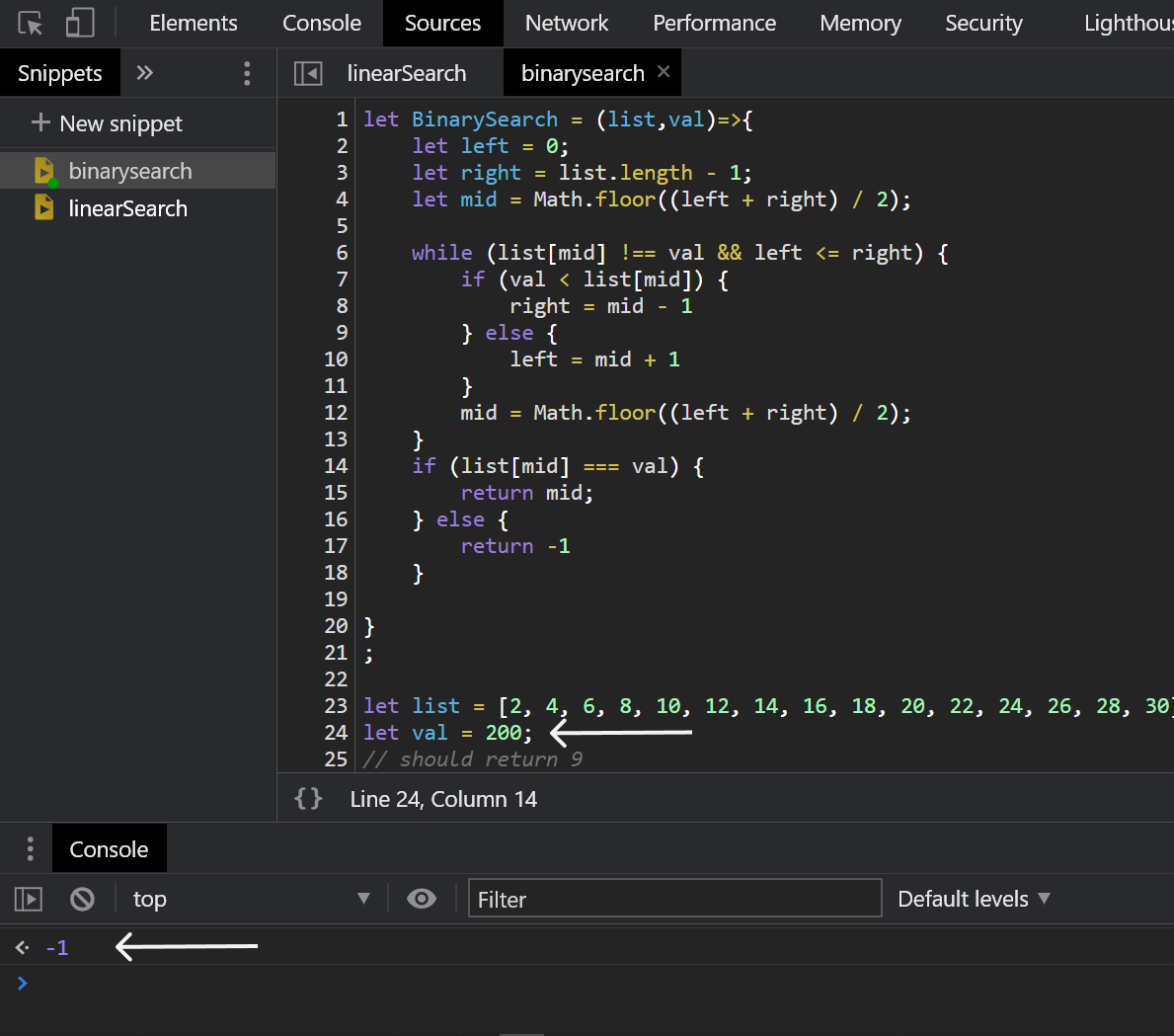# Conclusion

Both Binary Search and Linear Search has there own pros and cons. Linear Search loop through every item of the array which in large arrays would be less performant. But it works on all kinds of arrays. Binary Search on the other hand can be a lot faster but the downside to this algorithm is that it only works with sorted arrays.

# Get In Touch

Wanna get in touch or talk about a project?

Feel free to contact me via email at imran.ib@live.com